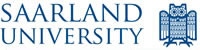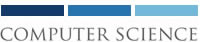# Tobias Tebbi: Master's Thesis Unification Modulo Nonnested Recursion Schemes via Anchored Semi-Unification## Abstract

A recursion scheme is an orthogonal rewriting system with rules of the form f(x₁,...,xₙ) → s. We consider terms to be equivalent if they rewrite to the same redex-free possibly infinite term after infinitary rewriting. For the restriction to the nonnested case, where nested redexes are forbidden, we prove the existence of principal unifiers modulo scheme equivalence. We give an algorithm computing principal unifiers by reducing the problem to a novel fragment of semi-unification we call anchored semi-unification. For anchored semi-unification, we develop a decision algorithm that returns a principal semi-unifier in the positive case and has a time complexity of O(n³α(n)) where α(n) is the inverse of the Ackermann function.